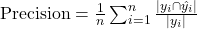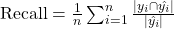###### Understanding Micro, Macro, and Weighted Averages for Scikit-Learn metrics in multi-class classification with example
2022-06-19###### A tutorial on Pandas apply, applymap, map, and transform
2022-06-23

In a traditional classification problem formulation, classes are mutually exclusive. In other words, under the condition of mutual exclusivity, each training example can belong only to one class. In such cases, classification errors occur due to overlapping classes in the feature space. However, often we encounter tasks where a data point can belong to multiple classes. In such cases, we pivot the traditional classification problem formulation to a Multi-Label Classification framework where we assume each label to be a Bernoulli random variable representing a different classification task.

Definitions:

• Multiclass classification: classification task with more than two classes. Each sample can only be labeled as one class.
• For example, classification using features extracted from a set of images of fruit, where each image may either be of an orange, an apple, or a pear. Each image is one sample and is labeled as one of the 3 possible classes. Multiclass classification makes the assumption that each sample is assigned to one and only one label – one sample cannot, for example, be both pear and an apple.
• Multilabel classification: classification task labeling each sample with x labels from n_classes possible classes, where x can be 0 to n_classes inclusive. This can be thought of as predicting properties of a sample that are not mutually exclusive. Formally, binary output is assigned to each class, for every sample. Positive classes are indicated with 1 and negative classes with 0 or -1. It is thus comparable to running n_classes binary classification tasks, for example with sklearn. multioutput.MultiOutputClassifier. This approach treats each label independently whereas multilabel classifiers may treat the multiple classes simultaneously, accounting for correlated behavior among them.
• For example, prediction of the topics relevant to a text document or video. The document or video may be about one of ‘religion’, ‘politics’, ‘finance’ or ‘education’, several of the topic classes, or all of the topic classes.

The difference between multi-class classification & multi-label classification is that in multi-class problems the classes are mutually exclusive, whereas for multi-label problems each label represents a different classification task, but the tasks are somehow related.

From an implementation standpoint –

1. We use the sigmoid activation function in the final layer instead of using a softmax activation.
2. We use binary cross-entropy loss instead of categorical cross-entropy.

There are numerous blogs out there with details on how to train a multi-label classifier using popular frameworks (Sklearn, Keras, Tensorflow, PyTorch, etc). However, evaluating the performance of any machine learning algorithm is a critical piece of the puzzle.

In this blog post, we would focus on different evaluation metrics that can be used for evaluating the performance of a multilabel classifier. The evaluation metrics for multi-label classification can be broadly classified into two categories —

• Example-Based Evaluation Metrics
• Label Based Evaluation Metrics.

## Example-Based Evaluation Metrics

The example-based evaluation metrics are designed to compute the average difference between the true labels and the predicted labels for each training data point, averaged over all the training examples in the dataset.

### 1. Exact Match Ratio (EMR)

• The Exact Match Ratio evaluation metric extends the concept of the accuracy from the single-label classification problem to a multi-label classification problem.
• One of the drawbacks of using EMR is that it does not account for partially correct labels.
def emr(y_true, y_pred):
n = len(y_true)
row_indicators = np.all(y_true == y_pred, axis = 1) # axis = 1 will check for equality along rows.
exact_match_count = np.sum(row_indicators)
return exact_match_count/n


## 1/0 Loss

• 1/0 Loss is basically (1 — EMR)
  def one_zero_loss(y_true, y_pred):
n = len(y_true)
row_indicators = np.logical_not(np.all(y_true == y_pred, axis = 1)) # axis = 1 will check for equality along rows.
not_equal_count = np.sum(row_indicators)
return not_equal_count/n


## Hamming Loss

• Hamming Loss computes the proportion of incorrectly predicted labels to the total number of labels.
• For a multilabel classification, we compute the number of False Positives and False Negative per instance and then average it over the total number of training instances.
def hamming_loss(y_true, y_pred):
"""
XOR TT for reference -

A  B   Output

0  0    0
0  1    1
1  0    1
1  1    0
"""
hl_num = np.sum(np.logical_xor(y_true, y_pred))
hl_den = np.prod(y_true.shape)

return hl_num/hl_den

hl_value = hamming_loss(y_true, y_pred)
print(f"Hamming Loss: {hl_value}")


## Example-Based Accuracy

• Accuracy for a training instance is defined as the proportion of predicted correct labels to the total number of labels for that training instance.
• The overall accuracy is the average accuracy across training instances.
def example_based_accuracy(y_true, y_pred):

# compute true positives using the logical AND operator
numerator = np.sum(np.logical_and(y_true, y_pred), axis = 1)

# compute true_positive + false negatives + false positive using the logical OR operator
denominator = np.sum(np.logical_or(y_true, y_pred), axis = 1)
instance_accuracy = numerator/denominator

avg_accuracy = np.mean(instance_accuracy)
return avg_accuracy

ex_based_accuracy = example_based_accuracy(y_true, y_pred)
print(f"Example Based Accuracy: {ex_based_accuracy}")


## Example-Based Precision

• Example-based precision is defined as the proportion of predicted correct labels to the total number of predicted labels, averaged over all instances.
def example_based_precision(y_true, y_pred):
"""
precision = TP/ (TP + FP)
"""

# Compute True Positive
precision_num = np.sum(np.logical_and(y_true, y_pred), axis = 1)

# Total number of pred true labels
precision_den = np.sum(y_pred, axis = 1)

# precision averaged over all training examples
avg_precision = np.mean(precision_num/precision_den)

return avg_precision

ex_based_precision = example_based_precision(y_true, y_pred)
print(f"Example Based Precision: {ex_based_precision}")


## Label Based Metrics

As opposed to example-based metrics, Label based metrics evaluate each label separately and then averaged over all labels. As a result, any metric that can be used for binary classification can be used as a label-based metric. These metrics can be computed on individual class labels and then averaged over all classes. This is termed Macro Averaging. Alternatively, we can compute these metrics globally over all instances and all class labels. This is termed Micro averaging.

Precision

It is the proportion of predicted correct labels to the total number of actual labels, averaged over all instances.def Precision(y_true, y_pred):
temp = 0
for i in range(y_true.shape):
if sum(y_true[i]) == 0:
continue
temp+= sum(np.logical_and(y_true[i], y_pred[i]))/ sum(y_true[i])
return temp/ y_true.shape
#0.5

• Recall: It is the proportion of predicted correct labels to the total number of predicted labels, averaged over all instances.def Recall(y_true, y_pred):
temp = 0
for i in range(y_true.shape):
if sum(y_pred[i]) == 0:
continue
temp+= sum(np.logical_and(y_true[i], y_pred[i]))/ sum(y_pred[i])
return temp/ y_true.shape
#0.375

• F1-Measure Definition of precision and recall naturally leads to the following definition for F1-measure (harmonic mean of precision and recall):def F1Measure(y_true, y_pred):
temp = 0
for i in range(y_true.shape):
if (sum(y_true[i]) == 0) and (sum(y_pred[i]) == 0):
continue
temp+= (2*sum(np.logical_and(y_true[i], y_pred[i])))/ (sum(y_true[i])+sum(y_pred[i]))
return temp/ y_true.shape

print(F1Measure(y_true, y_pred))
#0.41666666666666663


### Macro Averaged Accuracy

def label_based_macro_accuracy(y_true, y_pred):

# axis = 0 computes true positives along columns i.e labels
l_acc_num = np.sum(np.logical_and(y_true, y_pred), axis = 0)

# axis = 0 computes true postive + false positive + false negatives along columns i.e labels
l_acc_den = np.sum(np.logical_or(y_true, y_pred), axis = 0)

# compute mean accuracy across labels.
return np.mean(l_acc_num/l_acc_den)

lb_macro_acc_val = label_based_macro_accuracy(y_true, y_pred)
print(f"Label Based Macro Accuracy: {lb_macro_acc_val}")


### Macro Averaged Precision

def label_based_macro_precision(y_true, y_pred):

# axis = 0 computes true positive along columns i.e labels
l_prec_num = np.sum(np.logical_and(y_true, y_pred), axis = 0)

# axis = computes true_positive + false positive along columns i.e labels
l_prec_den = np.sum(y_pred, axis = 0)

# compute precision per class/label
l_prec_per_class = l_prec_num/l_prec_den

# macro precision = average of precsion across labels.
l_prec = np.mean(l_prec_per_class)
return l_prec

lb_macro_precision_val = label_based_macro_precision(y_true, y_pred)

print(f"Label Based Precision: {lb_macro_precision_val}")


### Macro Averaged Recall

def label_based_macro_recall(y_true, y_pred):

# compute true positive along axis = 0 i.e labels
l_recall_num = np.sum(np.logical_and(y_true, y_pred), axis = 0)

# compute true positive + false negatives along axis = 0 i.e columns
l_recall_den = np.sum(y_true, axis = 0)

# compute recall per class/label
l_recall_per_class = l_recall_num/l_recall_den

# compute macro averaged recall i.e recall averaged across labels.
l_recall = np.mean(l_recall_per_class)
return l_recall

lb_macro_recall_val = label_based_macro_recall(y_true, y_pred)
print(f"Label Based Recall: {lb_macro_recall_val}")


### Micro Averaged Accuracy

def label_based_micro_accuracy(y_true, y_pred):

# sum of all true positives across all examples and labels
l_acc_num = np.sum(np.logical_and(y_true, y_pred))

# sum of all tp+fp+fn across all examples and labels.
l_acc_den = np.sum(np.logical_or(y_true, y_pred))

# compute mirco averaged accuracy
return l_acc_num/l_acc_den

lb_micro_acc_val = label_based_micro_accuracy(y_true, y_pred)
print(f"Label Based Micro Accuracy: {lb_micro_acc_val}")


### Micro Averaged Precision

def label_based_micro_precision(y_true, y_pred):

# compute sum of true positives (tp) across training examples
# and labels.
l_prec_num = np.sum(np.logical_and(y_true, y_pred))

# compute the sum of tp + fp across training examples and labels
l_prec_den = np.sum(y_pred)

# compute micro-averaged precision
return l_prec_num/l_prec_den

lb_micro_prec_val = label_based_micro_precision(y_true, y_pred)
print(f"Label Based Micro Precision: {lb_micro_prec_val}")


### Micro Averaged Recall

# Function for Computing Label Based Micro Averaged Recall
# for a MultiLabel Classification problem.

def label_based_micro_recall(y_true, y_pred):

# compute sum of true positives across training examples and labels.
l_recall_num = np.sum(np.logical_and(y_true, y_pred))
# compute sum of tp + fn across training examples and labels
l_recall_den = np.sum(y_true)

# compute mirco-average recall
return l_recall_num/l_recall_den

lb_micro_recall_val = label_based_micro_recall(y_true, y_pred)
print(f"Label Based Micro Recall: {lb_micro_recall_val}")


### α- Evaluation Score

• Boutell et. al. in Learning multi-label scene classification introduced a generalized version of Jaccard Similarity for evaluating each multi-label prediction.
• The α-evaluation score provides a flexible way to evaluate multi-label classification results for both aggressive as well as conservation tasks.
def alpha_evaluation_score(y_true, y_pred):
alpha = 1
beta = 0.25
gamma = 1

# compute true positives across training examples and labels
tp = np.sum(np.logical_and(y_true, y_pred))

# compute false negatives (Missed Labels) across training examples and labels
fn = np.sum(np.logical_and(y_true, np.logical_not(y_pred)))

# compute False Positive across training examples and labels.
fp = np.sum(np.logical_and(np.logical_not(y_true), y_pred))

# Compute alpha evaluation score
alpha_score = (1 - ((beta * fn + gamma * fp ) / (tp +fn + fp + 0.00001)))**alpha

return alpha_score


One can also use Scikit Learn’s functions to compute accuracy, Hamming loss, and other metrics:

import sklearn.metrics

print('Exact Match Ratio: {0}'.format(sklearn.metrics.accuracy_score(y_true, y_pred, normalize=True, sample_weight=None)))
#Exact Match Ratio: 0.25

print('Hamming loss: {0}'.format(sklearn.metrics.hamming_loss(y_true, y_pred)))
#Hamming loss: 0.4166666666666667

#"samples" applies only to multilabel problems. It does not calculate a per-class measure, instead calculating the metric over the true and predicted classes
#for each sample in the evaluation data, and returning their (sample_weight-weighted) average.

print('Recall: {0}'.format(sklearn.metrics.precision_score(y_true=y_true, y_pred=y_pred, average='samples')))
#Recall: 0.375

print('Precision: {0}'.format(sklearn.metrics.recall_score(y_true=y_true, y_pred=y_pred, average='samples')))
#Precision: 0.5

print('F1 Measure: {0}'.format(sklearn.metrics.f1_score(y_true=y_true, y_pred=y_pred, average='samples')))
#F1 Measure: 0.41666666666666663


Here is a complete Jupyter Notebook on these metrics in scikit-learn.

Training a multi-label classification problem seems trivial with the use of abstract libraries. However, evaluating performance is a whole different ball game. Apart from evaluation metrics, computing and visualizing the confusion matrix for the Multi-label classification problem seems like another fun challenge.